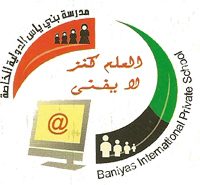Contact Us : 02/5827555 || Fax:: 02/5827222 || Email: info@adbips.com || Location of the school: Abu Dhabi - Baniyas - East 1#### School Policies And Regulations

##### Upcoming events

The Math skills required for the SAT are of a basic standard that should be within the reach of a tenth grade student. You don't need to learn up lots of new formulae but you will need to sharpen up your thinking skills.

The questions on the Math section are one of the following types:

I. Multiple Choice Questions
II. Student Response Questions or Grid In Questions
III. Math Practice Tests

The Math portion of the SAT is composed of three sections: two 25 minute sections and one 20 minute section. The following table summarizes the 3 math sections on the SAT:

Section Total Time Total Number of Questions
Section1 25 20 Multiple Choice Questions
Section2 25 8 Multiple Choice Questions
10 Student Response Questions
Section3 20 16 Multiple Choice questions

SAT Math Skills

The Math skills tested by the SAT are surprisingly limited; most of what is on the test has been covered by tenth grade. In addition to actual mathematical knowledge, many useful tricks and techniques can be used to quickly improve a student's score. Following is an overview of areas tested in Math section of the SAT:

1. Number and Operations (Approximately 10-14% of questions)

- Basic properties of numbers and their terminology (e.g. negative numbers, prime numbers, factors, integers, sets, sequences)
- Squares, square roots and exponents
- Order of Operations
- Fractions and decimals
- Percents
- Ratios and Proportions
- Averages
- Arithmetic Word Problems
Ā
2. Algebra and Functions (Approximately 38-42% of questions)

- Simplifying algebraic expressions
- Operations on Algebraic expressions (including factoring of quadratic equations)
- Equations and inequalities involving roots, exponents and absolute values
- Solving systems of equations and inequalities
- Direct and inverse variation
- Algebraic word problems
- Functions (domain, range, translations of graphs, functions using special symbols)
- Equations of lines (slope, intercept)
Ā
3. Geometry and Measurement (Approximately 38-42% of questions)
Ā
- Properties of parallel and perpendicular lines
- Coordinate Geometry (slopes, distance between points, midpoints of lines)
- Triangles (area, angles, properties of equilateral, isosceles, right and special triangles, the congruency and similarity of triangles, Pythagorean theorem)
- Quadrilaterals and other polygons (including area, interior and exterior angles, perimeters)
- Circles (area, circumference)
- Solid geometry (volume and surface area of solids)
- Transformations (translations, rotations, reflections)

4. Data Analysis, Statistics, and Probability (Approximately 6-10% of questions)
Ā
- Data interpretation (reading tables and line, bar and other graphs)
- Descriptive statistics (mean, median, mode, weighted average)
- Probability (of one event or two or more independent or dependent events)
- Geometric Probability (probability that a random point chosen will fall within a particular geometric figure)__________

It is important to try full-length practice tests under timed conditions; for this we recommend the tests in the Official SAT Study Guide.